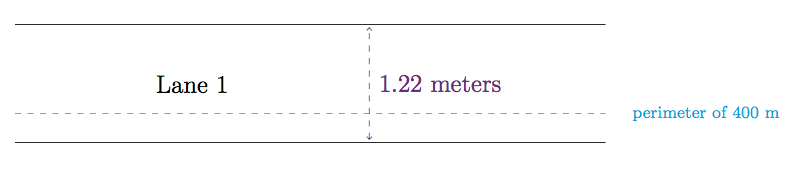# Running around a track I

Alignments to Content Standards: G-MG.A

An Olympic $400$ meter track is made up of two straight sides, each measuring $84.39$ meters in length, and two semi-circular curves with a radius of $36.5$ meters as pictured below:The picture is drawn to scale with one centimeter in the picture representing $20$ meters on an actual olympic track. The one of the eight lanes which is closest to the center of the track is called the first lane.

1. What is the perimeter of the track, measured on the innermost part of the first lane?
2. Each lane on the track is $1.22$ meters wide. What is the perimeter of the track measured on the outermost part of the first lane?
3. In order to run the intended $400$ meters in a lap, how far away from the inside of the first lane would a runner need to be?

Below is an enlarged picture of one of the straight sections of the track with the blue line representing the line around the track with perimeter exactly $400$ meters:## IM Commentary

In this problem geometry is applied to a $400$ meter track. The specifications for building a track for the Olympics are very precise and are laid out on pages $14$ and $15$ of

This task uses geometry to find the perimeter of the track. Students may be surprised when their calculation does not give $400$ meters but rather a smaller number. The teacher may wish to stop students at this point for a discussion of how this can be. Hopefully students will have the idea that if a runner's body were centered on the border of the track, the runner would only be half on the track and hence considered out of bounds. The next two parts of the problem give an idea where the runner needs to be in order to be running $400$ meters in one lap. If the students have a track at school they may want to measure out $30$ centimeters and see if it is realistic to run around the track with their body centered on this line.

This task is further developed in ''Running around a track II'' where the staggered starts for different lanes are considered. This task is ideal for instruction but could be use for assessment as well. If used for instruction purposes, the teacher may wish to ask students if the lane lines of the track are similar geometric shapes. This is a subtle question as the curved sections and the straight sections are similar but the entire shapes are not because there is a scale factor in the curves (with the outer lanes being radii of larger circles) while the straight sections are the same length for all lanes.

A simplified version of this task, without part (c) or with a different solution method, could be used in Grade 7 for 7.G.4, "Know the formulas for the area and circumference of a circle and use them to solve problems ...." And a more challenging modeling task for high school could remove some of the scaffolding and expect students to research the dimensions themselves.

## Solution

1. The two straightaway sections of the track are each $84.39$ meters. The two semi-circular sections can be joined to form a circle whose radius is $36.5$ meters and so the diameter of this circle is $2 \times 36.5 = 73$ meters. The circumference of this circle will be $\pi \times 73$ meters and so the total perimeter of the track is $$2 \times 84.39 + \pi \times 73 \approx 398.12.$$ So the perimeter of the track is less than $400$ meters.
2. For the first lane on the track, the straightaway sections are each $84.39$ meters long. However, the curved sections form a circle whose radius is now $36.5 + 1.22 = 37.72$ meters. The diameter of the circle will be $2 \times 37.72 = 75.44$ meters. So the perimeter of lane 1 is $$2 \times 84.39 + \pi \times 75.44 \approx 405.78.$$ So the perimeter of lane 1 on the track is more than $400$ meters and is almost $8$ meters more than the perimeter of the inside of the track.
3. Suppose we let $x$ denote the distance from the inside of lane 1 which gives a perimeter of $400$ meters. This perimeter will be consist of the two straight sections which contribute $2 \times 84.39$ meters to the perimeter. In addition, there will be two semi-circular sections of radius $36.5 + x$ meters. Combining these gives a circle whose diameter is $2 \times (36.5 + x)$ meters. So we want $$2 \times 84.39 + \pi \times 2 \times (36.5 + x) = 400.$$ Rewriting this we find $$2 \pi \times x = 400 - 2 \times 84.39 - 2\pi \times 36.5$$ Solving for $x$ we find $$x \approx 0.30.$$ Note that this value for $x$ is not exact but approximate. It is accurate to within about two ten thousandths of a meter or a fraction of a millimeter. So approximately $30$ centimeters from the inside of lane 1 the perimeter of the track is $400$ meters.